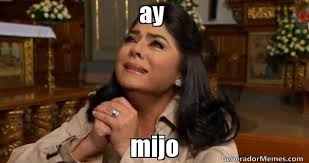# Mortgage formula and calculation

I realized the past CFA syllabus includes a LoS on mortgage interest calculation. I would like to learn the formula to calculate mortgage interest. Can anyone share the formula here or direct me to a site with a reliable formula? It seems like there are a lot of formulas out there and I’m unsure which is correct.

Dude, there is no formula for mortgage interest. Just take whatever your yearly interest rate is, divide it by 12 and multiply that by the balance on the loan for that periods interest payment.The BAII has loverly functions to calculate the total principal and interest payments between 2 dates.It’s wasaaay simpler than that bread man.

if you have a starting balance of 1 mill, and a monthly payment of 7,500. And if after two point five years you have a balance of only 800k, then everything over the \$200k in principal reduction is interest.

I don’t need calculator functions for that

Mijo, how did you get the \$800K figure??? What if I want to know what the principal and interest split is for the 73rd payment without calculating the outstanding principal???

Ay mijo…